Olympiad Test : Embedded Figure - 1

# Olympiad Test : Embedded Figure - 1

Test Description

## 20 Questions MCQ Test Maths Olympiad Class 6 | Olympiad Test : Embedded Figure - 1

Olympiad Test : Embedded Figure - 1 for Class 6 2022 is part of Maths Olympiad Class 6 preparation. The Olympiad Test : Embedded Figure - 1 questions and answers have been prepared according to the Class 6 exam syllabus.The Olympiad Test : Embedded Figure - 1 MCQs are made for Class 6 2022 Exam. Find important definitions, questions, notes, meanings, examples, exercises, MCQs and online tests for Olympiad Test : Embedded Figure - 1 below.
Solutions of Olympiad Test : Embedded Figure - 1 questions in English are available as part of our Maths Olympiad Class 6 for Class 6 & Olympiad Test : Embedded Figure - 1 solutions in Hindi for Maths Olympiad Class 6 course. Download more important topics, notes, lectures and mock test series for Class 6 Exam by signing up for free. Attempt Olympiad Test : Embedded Figure - 1 | 20 questions in 40 minutes | Mock test for Class 6 preparation | Free important questions MCQ to study Maths Olympiad Class 6 for Class 6 Exam | Download free PDF with solutions
 1 Crore+ students have signed up on EduRev. Have you?
Olympiad Test : Embedded Figure - 1 - Question 1

### Analyze the set of figures and choose the correct option that contains figure X.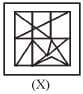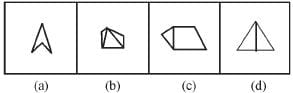Olympiad Test : Embedded Figure - 1 - Question 2

### Analyze the set of figures and choose the correct option that contains figure X.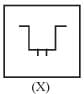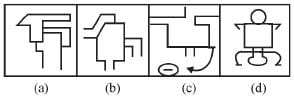Olympiad Test : Embedded Figure - 1 - Question 3

### Analyze the set of figures and choose the correct option that contains figure X.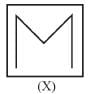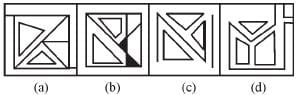Olympiad Test : Embedded Figure - 1 - Question 4

Analyze the set of figures and choose the correct option that contains figure X.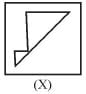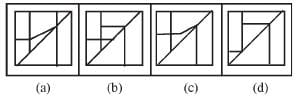Olympiad Test : Embedded Figure - 1 - Question 5

Analyze the set of figures and choose the correct option that contains figure X.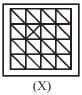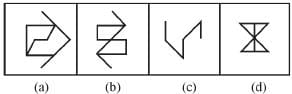Olympiad Test : Embedded Figure - 1 - Question 6

Analyze the set of figures and choose the correct option that contains figure X.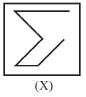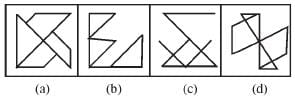Olympiad Test : Embedded Figure - 1 - Question 7

Analyze the set of figures and choose the correct option that contains figure X.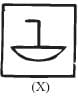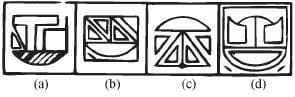Olympiad Test : Embedded Figure - 1 - Question 8

Analyze the set of figures and choose the correct option that contains figure X.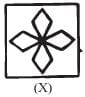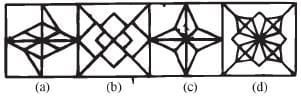Olympiad Test : Embedded Figure - 1 - Question 9

Analyze the set of figures and choose the correct option that contains figure X.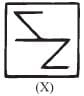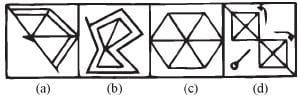Olympiad Test : Embedded Figure - 1 - Question 10

Analyze the set of figures and choose the correct option that contains figure X.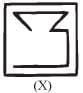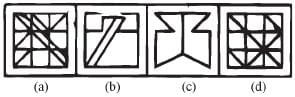Olympiad Test : Embedded Figure - 1 - Question 11

Analyze the set of figures and choose the correct option that contains figure X.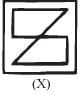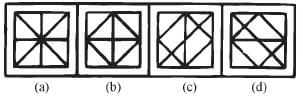Olympiad Test : Embedded Figure - 1 - Question 12

Analyze the set of figures and choose the correct option that contains figure X.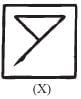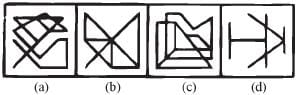Olympiad Test : Embedded Figure - 1 - Question 13

Analyze the set of figures and choose the correct option that contains figure X.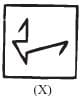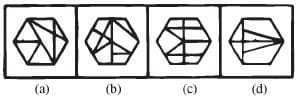Olympiad Test : Embedded Figure - 1 - Question 14

Analyze the set of figures and choose the correct option that contains figure X.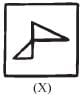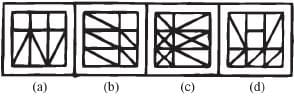Olympiad Test : Embedded Figure - 1 - Question 15

Analyze the set of figures and choose the correct option that contains figure X.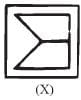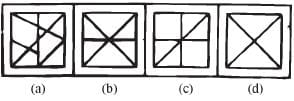Olympiad Test : Embedded Figure - 1 - Question 16

Analyze the set of figures and choose the correct option that contains figure X.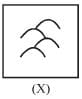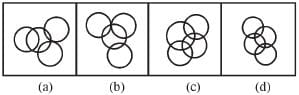Olympiad Test : Embedded Figure - 1 - Question 17

Analyze the set of figures and choose the correct option that contains figure X.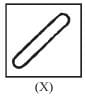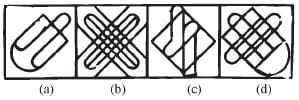Olympiad Test : Embedded Figure - 1 - Question 18

Analyze the set of figures and choose the correct option that contains figure X.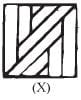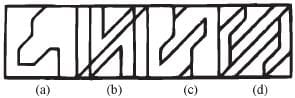Olympiad Test : Embedded Figure - 1 - Question 19

Analyze the set of figures and choose the correct option that contains figure X.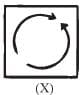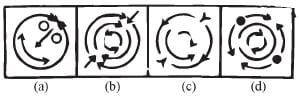Olympiad Test : Embedded Figure - 1 - Question 20

Analyze the set of figures and choose the correct option that contains figure X.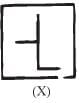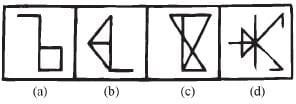## Maths Olympiad Class 6

43 tests
 Use Code STAYHOME200 and get INR 200 additional OFF Use Coupon Code
Information about Olympiad Test : Embedded Figure - 1 Page
In this test you can find the Exam questions for Olympiad Test : Embedded Figure - 1 solved & explained in the simplest way possible. Besides giving Questions and answers for Olympiad Test : Embedded Figure - 1, EduRev gives you an ample number of Online tests for practice

43 tests